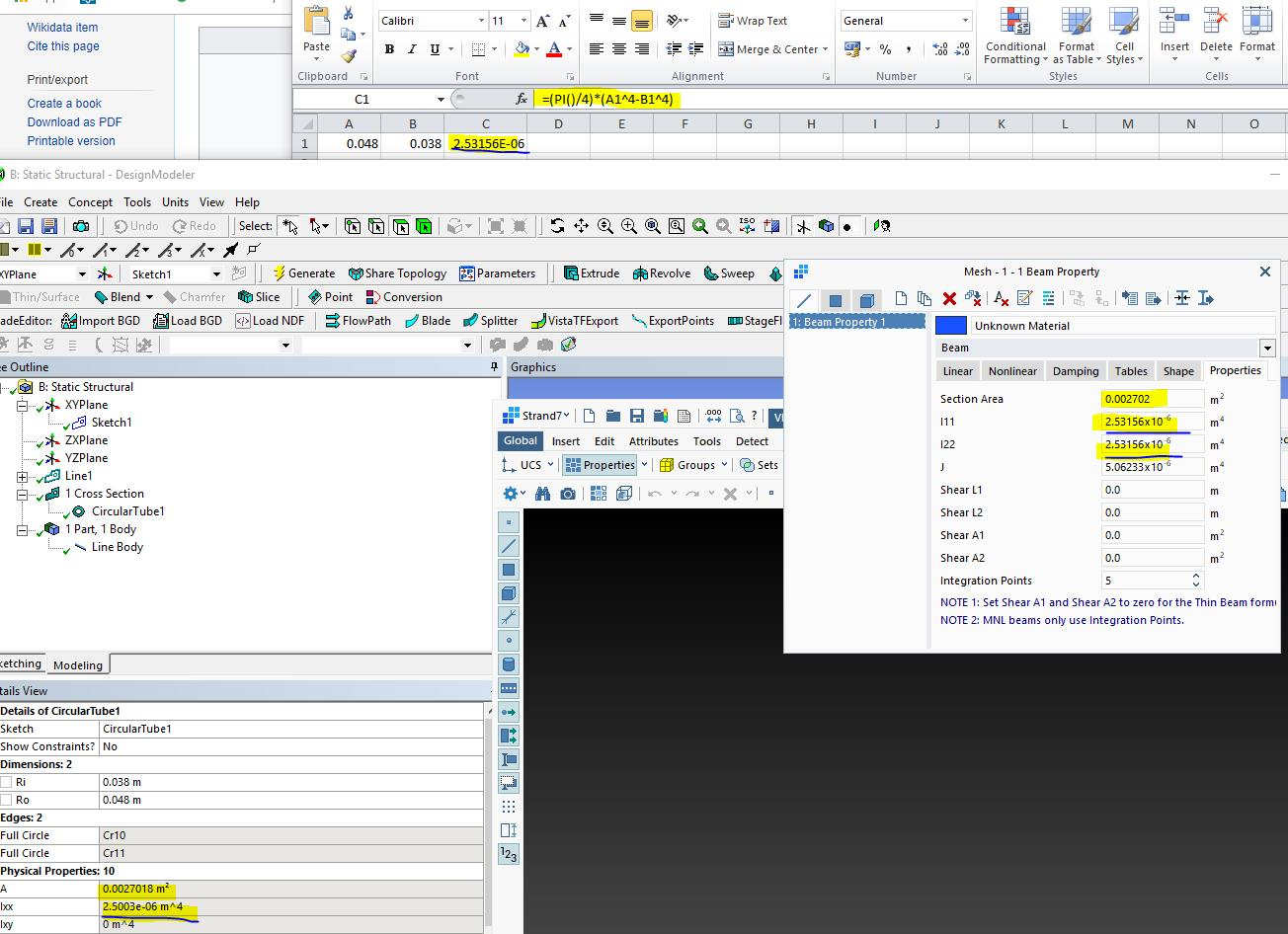## General Mechanical

#### fixed both end beam subject to bending – hand calculation is diferent from simulation result

•bigblue100
Subscriber

Hi there,

I have a beam with both ends fixed on the wall. (please refer to attached image) The length is 2.63m, and cross section of beam is pipe with inner and outer diameter of 0.048m and 0.38 respectively. In ANSYS, I have drew up the model with line body, assign the cross section to line, set the boundary condition as fixed at both ends and meshed with 0.001m element size. I have the simulation results of reaction force and moment the same to what I have calculated by hand, but the maximum deflection, 0.8mm from simulation, is far different from 12mm by hand calculation. The equation I used to calculate the deflection is qL^4/384EI, where E=2x10^11, I=1.6x10^-7. I used the default structural steel as material and line pressure as a distributed force.

I have double checked all the parameters inputted into ANSYS, but they all looks correct. I have also checked all the equations I used to do the hand calculation.

•peteroznewman
Subscriber

The area moment of inertia of a pipe with an OD of .38 m and an ID of 0.048 m is 1.023x10^-3 m^4

You have the wrong value of 1.6x10^-7 m^4. There may be other mistakes, I stopped when I found one.

•bigblue100
Subscriber

Hi Peter,

I've just realised that I typed the wrong OD. OD is 0.048 and ID is 0.038.

I have checked within ANSYS and found the second moment of inertia was calculated as 2.5 x 10^-6 by the software. This figure is not right and I found I cannot correct it. I use the line body with circular profile assgined to. Please help and instruct.

Thank you.

•peteroznewman
Subscriber

Is the beam drawn in DesignModeler or SpaceClaim?

In either case, you can edit the circular profile to change the OD and ID to the correct values.

•bigblue100
Subscriber

Hi Peter,

The beam was drawn in the Design Modeler. I have put the OD and ID in, but the second moment of inertia is incorrect. As I described in my last message,I have checked everthing, but nothing seems wrong.

What else could be the problem?

•jj77
Subscriber

The value calculated by ANsys is correct (see below agrees with other software and hand calcs.)-your hand calcs for Ixx, and Iyy are wrong (see below in the excel formula for the correct equation for a tube - this is not a thin walled CHS).•bigblue100
Subscriber

Hi JJ77,

I was awear that there are two equations to calculate second moment of inertia on a cylinderical geometry. The one you used is for solid cylindrical section, but my model is a hollow section with a wall thickness of 5mm. So why do we have to use the solid section formula to calculate the hollow section? Please instruct.

Thank you.

•bigblue100
Subscriber

The equation I used to calculate is (PI()/64)*(R1^4-R2^4)

•bigblue100
Subscriber

Sorry JJ77, you are right. I just realised I used the radius instead of diameter as soon as I typed the equation in my previous reply.